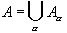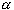#Interactive Real Analysis

Next | Previous | Glossary | Map

## 1.3. Equivalence Relations and Classes

### Theorem 1.3.3: Equivalence Classes

Let r be an equivalence relation on a set A. Then A can be written as a union of disjoint setswith the following properties:
1. If a,b are in A then a ~ b if and only if a and b are in the same set2. The subsetsare non-empty and pairwise disjoint.
The setsare called equivalence classes.

### Proof:

We have to decide what the equivalence classesshould be: Since by property (1) two elements a and b are supposed to be related if and only if they are in the same class, it seems natural to define:
•= { bA : b ~}
To simplify notation a little, we denote this class by A() . Now we need to check whether this is a good definition, and whether it satisfies the above properties.

Because of reflexivity of the equivalence relation, the class A(a) contains the element a and is therefore not empty.

Next, we will show that two classes that are different can not have any elements in common, i.e. they are disjoint. Recall that disjoint means that two sets have either an empty intersection or are the same sets. Take two elements a and a' and suppose that A(a) and A(a') have a non-empty intersection; say both classes contain the element c. Then

• c ~ a, because c is in A(a)
• a ~ c, by symmetry and the above line
• c ~ a' because c is in A(a')
• Therefore, by transitivity, a ~ a'
Now take any element b in A(a'). Then b ~ a' and a' ~ a, so that b ~ a by transitivity, and hence b is contained in A(a). Therefore A(a') is a subset of A(a). The same argument works the other way around as well (by symmetry), showing that A(a) is a subset of A(a'). Hence, A(a) = A(a'). This shows that two different classes must be disjoint.

Finally, we need to show that all classes A(a) make up the original set A. But that is clear, because every element a in A belongs to the class A(a), hence the union of all classes A(a) must be contained in A, and therefore must be equal to A.Next | Previous | Glossary | Map